# NCERT Solutions for Class 12 Physics Chapter 4 Moving Charges and Magnetism

In this chapter, we provide NCERT Solutions for Class 12 Physics Chapter 4 Moving Charges and Magnetism for English medium students, Which will very helpful for every student in their exams. Students can download the latest NCERT Solutions for Class 12 Physics Chapter 4 Moving Charges and Magnetism pdf, free NCERT solutions for Class 12 Physics Chapter 4 Moving Charges and Magnetism book pdf download. Now you will get step by step solution to each question. Class 12 physics is a very important subject for entrance exams like IIT JEE, CPMT, etc.

## NCERT Solutions for Class 12 Physics Chapter 4 Moving Charges And Magnetism

Question 1.
A circular coil of wire consisting of 100 turns, each of radius 8.0 cm carries a current of 0.4 A. What is the magnitude of the magnetic field 6 at the centre of the coil?
Solution:
The magnetic field at the centre of a circular coil having 100 turns.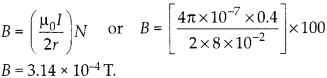Question 2.
A long straight wire carries a current of 35 A. What is the magnitude of the field 6 at a point 20 cm from the wire?
Solution:
Magnetic field due to a long straight wire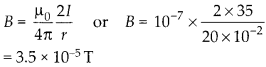Question 3.
A long straight wire in the horizontal plane carries a current of 50 A in north to south direction. Give the magnitude and direction of B at a point 2.5 m east of the wire.
Solution:
Let us first decide the standard directions on the plane of paper.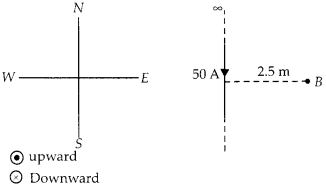Magnitude of magnetic field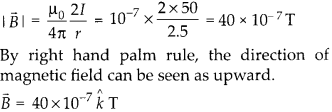Question 4.
A horizontal overhead power line carries a current of 90 A in east to west direction. What is the magnitude and direction of the magnetic field due to the current 1.5 m below the line?
Solution:
The standard directions on the plane of paper can be different according to requirements.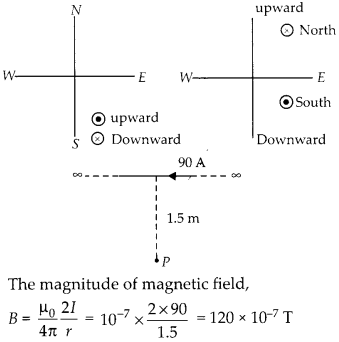Direction of magnetic field can be observed by right hand palm rule and it is southward.

Question 5.
What is the magnitude of magnetic force per unit length on a wire carrying a current of 8 A and making an angle of 30° with the direction of a uniform magnetic field of 0.15 T?
Solution: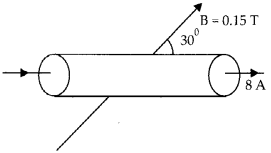Let l be length of wire, carrying a current of 8 A at an angle 30° with the magnetic field. Force on the wire, F = |B| sinθ Force per unit length F/l = |B| sinθ F/l = 8 × 0.15 × sin 30° = 0.6 N m-1.

Question 6.
A 3.0 cm wire carrying a current of 10 A is placed inside a solenoid perpendicular to its axis. The magnetic field inside the solenoid is given to be 0.27 T. What is the magnetic force on the wire?
Solution:
The magnetic field inside the solenoid is along its axis. the current in the wire flows perpendicular to the axis. F = |B| sin 90° or F = 10 × 0.27 × 3 × 10-2 x 1 = 0.081 N

Question 7.
Two long and parallel straight wires A and B carrying currents of 8.0 A and 5.0 A in the same direction are separated by a distance of 4.0 cm. Estimate the force on a 10 cm section of wire A.
Solution:
Force of attraction per unit length on two parallel wires carrying current in same direction.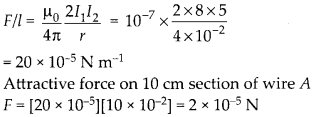Question 8.
A closely wound solenoid 80 cm long has 5 layers of windings of 400 turns each. The diameter of the solenoid is 1.8 cm. If the current the carried is 8.0 A, estimate the magnitude of 8 inside the solenoid near its centre.
Solution:
Total number of turns in 80 cm length of solenoid can be calculated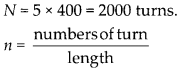Question 9.
A square coil of side 10 cm consists of 20 turns and carries a current of 12 A. The coil is suspended vertically and the normal to the plane of the coil makes an angle of 30° with the direction of a uniform horizontal magnetic field of magnitude 0.80 T. What is the magnitude of torque experienced by the coil?
Solution: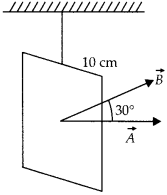Torque experienced by the coil carrying current in the given magnetic field.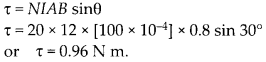Question 10.
Two moving coil meters, M1 and M2 have following particulars: R1 = 10Ω, N1 = 30, A1 = 3.6 × 10-3 m2, B1 = 0.25 T R2 = 14 Ω, N2 = 42, A2 = 1.8 × 10-3 m2, B2 = 0.50 T. (The spring constants are identical for two meters). Determine ratio of (i) current sensitivity (ii) voltage sensitivity of M2 and A4,.
Solution:
Current sensitivity of a moving coil galvanometer is defined as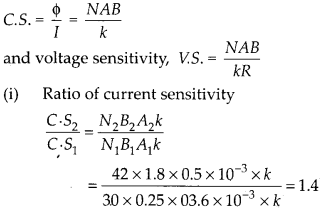(ii) Ratio of voltage sensitivity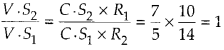Question 11.
In a chamber, a uniform magnetic field of 6.5 G (1 G = 10-4 T) is maintained. An electron is shot into the field with a speed of 4.8 × 106 m s-1 normal to the field. Explain why the path of the electron is a circle. Determine the radius of the circular orbit. (e= 1.6 × 10-19 C,me = 9.1 × 10-31 kg)
Solution:
The magnetic force ƒ = quB act normal to the direction of motion, thus provide the necessary centripetal force to follow the circular path.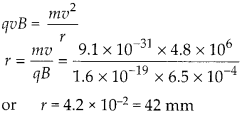Question 12.
In question 11, obtain the frequency of revolution of the electron in its circular orbit. Does the answer depend on the speed of the electron? Explain.
Solution:
Time period can be calculated.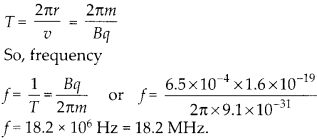The frequency of revolution of electron is independent of speed of electron.

Question 13.
(a) A circular coil of 30 turns and radius 8.0 cm carrying a current of 6.0 A is suspended vertically in a uniform horizontal magnetic field of magnitude 1.0 T. The field lines make an angle of 60° with the normal of the coil. Calculate the magnitude of the counter torque that must be applied to prevent the coil from turning.
(b) Would your answer change, if the circular coil in (a) were replaced by a planar coil of some irregular shape that encloses the same area? [All other particulars are also unaltered].
Solution:
(a) The given coil is circular and is suspended such that field lines makes angle 60° with normal of the coil.A similar torque is required to prevent the coil from turning.A similar torque is required to prevent the coil from turning.
(b) As long as the area of the planar coil remains same, the torque on the coil is also same, irrespective of the shape.

Question 14.
Two concentric circular coils X and Y of radii 16 cm and 10 cm, respectively, lie in the same vertical plane containing the north to south direction. Coil X has 20 turns and carries a current of 16 A; coil Y has 25 turns and carries a current of 18 A. The sense of the current in X is anticlockwise, and clockwise in Y, for an observer looking at the coils facing west. Give the magnitude and direction of the net magnetic field due to the coils at their centre.
Solution:
The concentric coils are in the plane north to south. Let us decide the directions with conveniences in the plane of paper.Magnetic field at centre due to coil X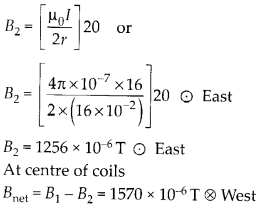So, a magnetic field nearly 1.6 × 10-3 T will appear at centre of the circular coil directed towards west.

Question 15.
A magnetic field of 100 G (1G = 10-4 T) is required which is uniform in a region of linear dimension about 10 cm and area of cross-section about 103-3 m2. The maximum current-carrying capacity of a given coil of wire is 15 A and the number of turns per unit length that can be wound round a core is at most 1000 turns m”1. Suggest some appropriate design particulars of a solenoid for the required purpose. Assume the core is not ferromagnetic.
Solution:
The magnetic field required is 100 G in a length of 10 cm. So, the solenoid should have a length larger than 10 cm. The maximum current capacity of wire is 15 A, so less than 15 A should flow in wire. Now using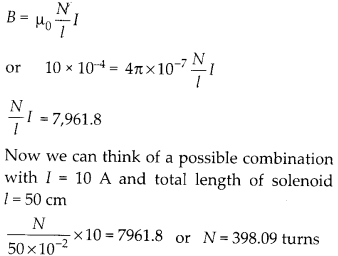So, one combination for the desired 100 G magnetic field in a length 10 cm can be a total length of solenoid of 50 cm and current in the solenoid of 10 A and total turns nearly 400.

Question 16.
Fora circular coil of radius R and N turns carrying current I, the magnitude of the magnetic field at a point on its axis at a distance x from its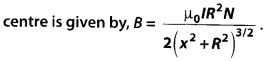(a) Show that this reduces to the familiar result for field at the centre of the coil
(b) Consider two parallel co-axial circular coils of equal radius R, and number of turns N, carrying equal currents in the same direction, and separated by a distance R. Show that the field on the axis around the mid-point between the coils is uniform over a distance that is small as compared to R, and is given by,[Such an arrangement to produce a nearly uniform magnetic field over a small region is known as Helmholtz coils.]
Solution:
On the axis of the circular coil of radius R and N turns carrying a current I at a distance x from centre the magnetic field is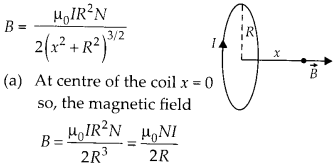Which is the same result as is obtained by integration at centre of the coil.
(b) The side of the loop where current flows clockwise becomes south pole and where the current appear anticlockwise becomes north pole. Direction of magnetic field is from south pole to north pole.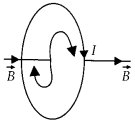Now, two parallel coils each of radius R with N turns. The magnetic field of both the coils add.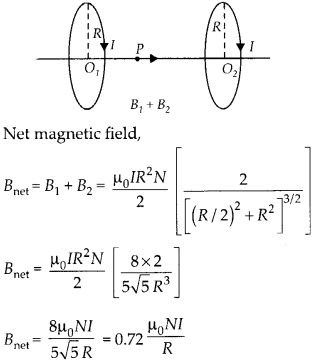Question 17.
A toroid has a core (non-ferromagnetic) of inner radius 25 cm and outer radius 26 cm, around which 3500 turns of a wire are wound. If the current in the wire is 11 A, what is the magnetic field
(a) outside the toroid,
(b) inside the core of the toroid, and
(c) in the empty space surrounded by the toroid.
Solution: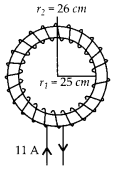Total turns in the toroid are given 3500.
(a) outside the toroid the magnetic field is zero.
(b) Inside the core of toroid, the magnetic field will be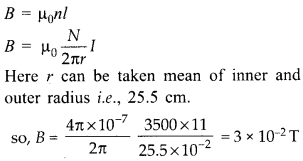(c) Magnetic field in the empty space surrounded by the toroid is also zero.

Question 18.
(a) A magnetic field that varies in magnitude from point to point but has a constant direction (east to west) is set up in a chamber. A charged particle enters the chamber and travels undeflected along a straight path with constant speed. What can you say about the initial velocity of the particle?
(b) A charged particle enters an environment of a strong and non-uniform magnetic field varying from point to point both in magnitude and direction, and comes out of it following a complicated trajectory. Would its final speed equal the initial speed if it suffered no collisions with the environment?
(c) An electron travelling west to east enters a chamber having a uniform electrostatic field in north to south direction. Specify the direction in which a uniform magnetic field should be set up to prevent the electron from deflecting from its straight line path.
Solution:
(a) If a charged particle move parallel or anti parallel to the magnetic field, no magnetic force will act on it and it move undeflected. So, in the given condition either the charged particle enters east to west or west to east.
(b) A magnetic force can only change the direction of charged particle but never changes magnitude of speed as force act normal to direction of speed. So, charged particle may follow a complicated trajectory, but its speed remains the same.
(c)If,we want the electron to move undeflected in the presence of electric and magnetic fields, then the electric force should be balanced by magnetic force.So, the magnetic field should act in downward direction.

Question 19.
An electron emitted by a heated cathode and accelerated through a potential difference of 2.0 kV, enters a region with uniform magnetic field of 0.15 T. Determine the trajectory of the electron if the field (a) is transverse to its initial velocity, (b) makes an angle of 30° with the initial velocity.
Solution:
A electron which is accelerated by a potential difference of 2.0 kV will have a kinetic energy gained 2000 eV.(a) When the electron enters in the uniform r magnetic field which is normal to the velocity of electron the electron follows a circular path r of radius.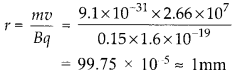(b) When the magnetic field makes an angle 30° with the initial velocity, the trajectory of the electron becomes helical. radius of the helical path is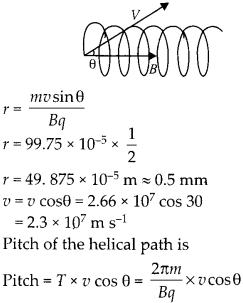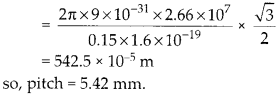Question 20.
A magnetic field set up using Helmholtz coils (described in question 16 above) is uniform in a small region and has a magnitude of 0.75 T. In the same region, a uniform electrostatic field is maintained in a direction normal to the common axis of the coils. A narrow beam of (single species) charged particles all accelerated through 15 kV enters this region in a direction perpendicular to both the axis of the coils and the electrostatic field. If the beam remains undeflected when the electrostatic field is 9.0 × 10-5 V m-1, make a simple guess as to what the beam contains. Why is the answer not unique?
Solution:
Narrow beam of charged particles remains undeflected and is perpendicular to both electric field and magnetic fields which are mutually perpendicular. So, the electric force is balanced by magnetic force. qE = quB Speed of charged particlesBecause the beam is accelerated through 15 kV, if charge is q, then kinetic energy gained by charged particles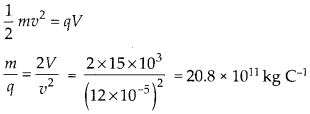Here, we can only obtain charge to mass ratio and same ratio can be in Deuterium ions, He++, Li+++, so the beam can contain any of these charged particles.

Question 21.
A straight horizontal conducting rod of length 0.45 m and mass 60 g is suspended by two vertical wires at its ends. A current of 5.0 A is set up in the rod through the wires.
(a) What magnetic field should be set up normal to the conductor in order that the tension in the wires is zero?
(b) What will be the total tension in the wires if the direction of current is reversed keeping the magnetic field same as before?
[Ignore the mass of the wires.] g = 9.8 m s-2
Solution:

Tension in the strings and magnetic force |B| balance the weight of wire. 2 T + |B| = mgQuestion 22.
The wires which connect the battery of an automobile to its starting motor carry a current of 300 A (for a short time). What is the force per unit length between the wires if they are 70 cm long and 1.5 cm apart? Is the force attractive or repulsive?
Solution:
Two wires connecting battery of an automobile to the starting motor carry 300 A current in opposite direction. So, the force is repulsive between them. Force per unit length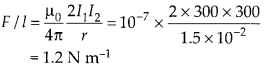Question 23.
A uniform magnetic field of 1.5 T exists in a cylindrical region of radius 10.0 cm, its direction parallel to the axis along east to west. A wire carrying current of 7.0 A in the north to south direction passes through this region. What is the magnitude and direction of the force on the wire if,
(a) the wire intersects the axis,
(b) the wire is turned from N-S to northeast- northwest direction.
(c) the wire in the N-S direction is lowered from the axis by a distance of 6.0 cm?
Solution: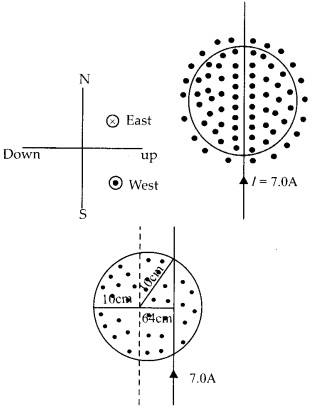The magnetic field is in the direction east to west and in the cylindrical region of radius 10 cm.
(a) A current carrying wire, intersects the axis. Force on wire, F = |B| = IB (2r) = 7 × 1.5 × 2 × 1 × 10-2 F = 2.1 N Downwards
(b) By turning the wire by an angle 45° in NE and NW direction the force remain the same,/= 2.1 N Downwards
(c) Now the wire is lowered from the axis by a distance of 6.0 cm.Length of wire inside cylindrical region is 16 cm now. So, the force is F = |B| = 7 × 1.5 × 16 × 10-2 = 1.68 N, vertically downwards

Question 24.
A uniform magnetic field of 3000 G is established along the positive z-direction. A rectangular loop of sides 10 cm and 5 cm carries a current of 12 A. What is the torque on the loop in the different cases shown in figure? What is the force on each case? Which case corresponds to stable equilibrium?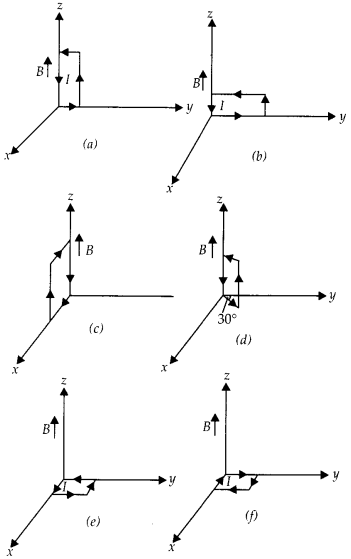Solution:
(a) Let us detail each case separately,So, torque is 1.8 × 10-2 N-m along -y direction net force on the coil is zero coil not in equilibrium.
(b) Dipole moment is along +x direction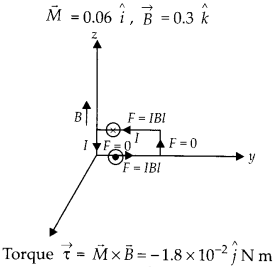So, torque is 1.8 x 10-2 N m along -y direction. Net force on the coil is zero, coil is not in equilibrium
(c) Dipole moment is along -y direction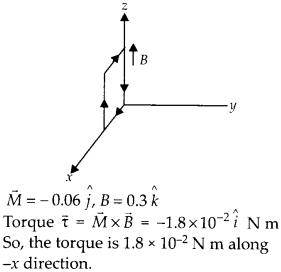Net force on the coil is zero, coil is not in equilibrium.
(d)

Dipole moment is at an angle 150° with the +x direction.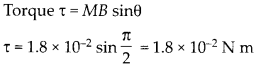At an angle 240° with the +x direction net force on the coil is zero, coil is not in equilibrium.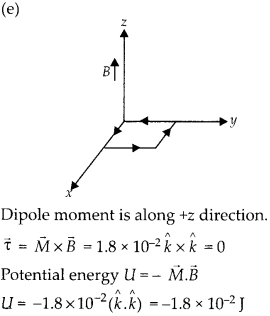Negative energy shows equilibrium is stable,
(f) Dipole moment is along – z direction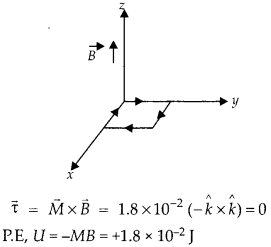Positive energy shows equilibrium is unstable.

Question 25.
A circular coil of 20 turns and radius 10 cm is place in a uniform magnetic field of 0.10 T normal to the plane of the coil. If the current in the coil is 5.0 A, what is the
(a) total torque on the coil,
(b) total force on the coil,
(c) average force on each electron in the coil due to the magnetic field?
(The coil is made of copper wire of cross-sectional area 10-5 m2, the free electron density in copper is given to be about 1029   m-3.)
Solution:
The magnetic field is normal to the plane of the coil, so condition of minimum torque.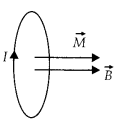(a) Torque on the coil τ = NIB A sinθ here θ = 0° τ = 0
(b) Force on every element of the coil is cancelled by force on corresponding element. Net force on the unit is zero.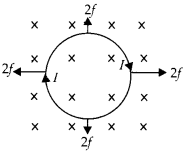(c) To calculate force on each electron, let us find drift velocity.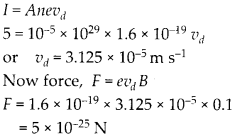Question 26.
A solenoid 60 cm long and of radius 4.0 cm has 3 layers of windings of 300 turns each. A 2.0 cm long wire of mass 2.5 g lies inside solenoid (near its centre) normal to its axis; both the wire and axis of the solenoid are in the horizontal plane. The wire is connected through two leads parallel to the axis of the solenoid to an external battery which supplies a current of 6.0 A in the wire. What value of current [with appropriate sense of circulation] in the windings of the solenoid can support the weight of the wire? g = 9.8 m s-2.
Solution:
Magnetic in the solenoid B = μ0nI

A current carrying wire is suspended inside solenoid and a current 7 = 6.0 A is flowing in it. To balance the weight of the wire by the magnetic force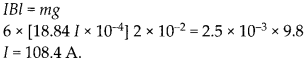Question 27.
A galvanometer coil has a resistance of 12 Q and the metre shows full scale deflection for a current of 3 mA. How will you convert metre into a voltmeter of range 0 to 18 V?
Solution:
By using the formulaWe can calculate required resistance to be connected in series.Question 28.
A galvanometer coil has a resistance of 15 Q and the meter shows full scale deflection for a current of 4 mA. How will you convert metre into an ammeter of range 0 to 6 A?
Solution:
For converting galvanometer into ammeter of required range, required shunt can be calculatedAll Chapter NCERT Solutions For Class12 Physics

—————————————————————————–

All Subject NCERT Solutions For Class12

*************************************************

Remark:

I think you got complete solutions for this chapter. If You have any queries regarding this chapter, please comment on the below section our subject teacher will answer you. We tried our best to give complete solutions so you got good marks in your exam.

If these solutions have helped you, you can also share Careerkundali.in to your friends.

Best of Luck!!